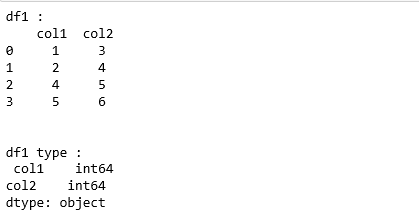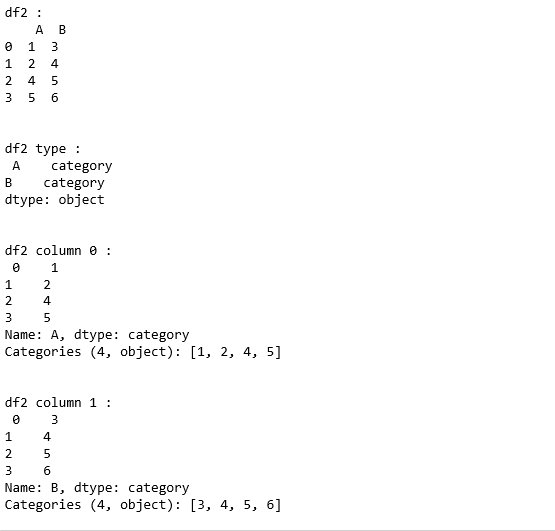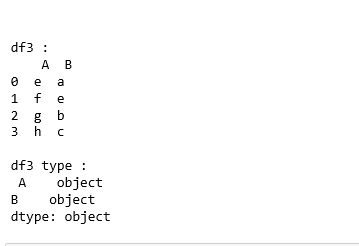Skip to content
Related Articles
Python | Pandas Categorical DataFrame creation
• Last Updated : 20 May, 2019

pandas.DataFrame(dtype=”category”) : For creating a categorical dataframe, dataframe() method has dtype attribute set to category.
All the columns in data-frame can be converted to categorical either during or after construction by specifying dtype=”category” in the DataFrame constructor.

Code :

 `# Python code explaining``# constructing categorical data frame``  ` `# importing libraries``import` `numpy as np``import` `pandas as pd`` ` `# Constructing dataframe ``data ``=` `{``'col1'``: [``1``, ``2``, ``4``, ``5``], ``'col2'``: [``3``, ``4``, ``5``, ``6``]}``df1 ``=` `pd.DataFrame(data ``=` `data)`` ` `print` `(``"df1 : \n"``, df1)``print``(``"\n\ndf1 type :\n"``, df1.dtypes)`

Output :`# Converting dataframe to category``df2 ``=` `pd.DataFrame({``'A'``: ``list``(``'1245'``), ``'B'``: ``list``(``'3456'``)}, dtype ``=``"category"``)`` ` `print` `(``"df2 : \n"``, df2)``print``(``"\n\ndf2 type :\n"``, df2.dtypes)`` ` `print` `(``"\n\ndf2 column 0 :\n"``, df2[``'A'``])``print` `(``"\n\ndf2 column 1 :\n"``, df2[``'B'``])`

Output :`# Conversion can be done using astype()``df3 ``=` `pd.DataFrame({``'A'``: ``list``(``'efgh'``), ``'B'``: ``list``(``'aebc'``)})``print` `(``"\n\ndf3 : \n"``, df3)``print``(``"\ndf3 type :\n"``, df3.dtypes)`` ` `df4 ``=` `df3.astype(``'category'``)``print` `(``"\n\ndf4 type:\n"``, df4.dtypes)`

Output :Attention geek! Strengthen your foundations with the Python Programming Foundation Course and learn the basics.

To begin with, your interview preparations Enhance your Data Structures concepts with the Python DS Course. And to begin with your Machine Learning Journey, join the Machine Learning – Basic Level Course

My Personal Notes arrow_drop_up